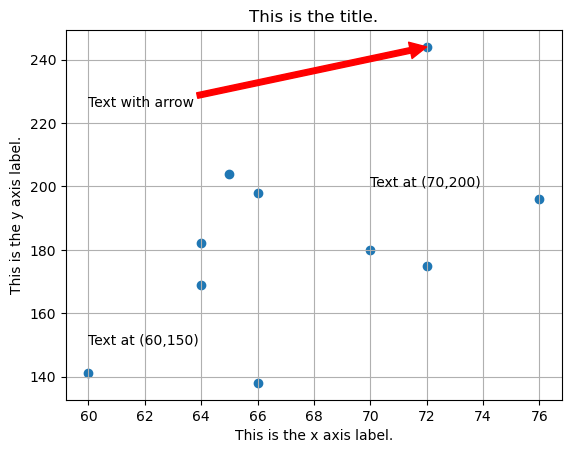# How to add details to a plot (in Python, using Matplotlib)

See all solutions.

After making a plot, we might want to add axis labels, a title, gridlines, or text. Plotting packages provide tons of tools for this sort of thing. What are some of the essentials?

Related topics:

## Solution

We will create some fake data using Python lists, for simplicity. But everything we show below works also if your data is in columns of a DataFrame, such as df['age'].

1
2
patient_height = [  60,  64,  64,  65,  66,  66,  70,  72,  72,  76 ]
patient_weight = [ 141, 182, 169, 204, 138, 198, 180, 175, 244, 196 ]


The conventional way to import matplotlib in Python is as follows.

1
import matplotlib.pyplot as plt


The following code creates a plot with many details added, but each is independent of the others, so you can take just the bit of code that you need.

1
2
3
4
5
6
7
8
9
10
plt.scatter( patient_height, patient_weight )
plt.xlabel( 'This is the x axis label.' )
plt.ylabel( 'This is the y axis label.' )
plt.title( 'This is the title.' )
plt.grid()                                     # Turns on gridlines
plt.text( 70, 200, 'Text at (70,200)' )        # Text method 1
plt.annotate( 'Text at (60,150)', (60,150) )   # Text method 2
plt.annotate( 'Text with arrow', xytext=(60,225), xy=(72,244),
arrowprops={'color':'red'} )     # Text with arrow
plt.show()